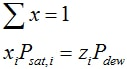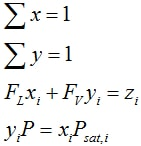In the Ideal Thermodynamic example, only the vapor and liquid phases are provided. Only a single liquid phase is supported. The following flash specifications are supported:

• Temperature and pressure
• Temperature and vapor phase fraction
• Pressure and vapor phase fraction
• Pressure and enthalpy
• Pressure and entropy

As the vapor phase enthalpy does not depend on pressure, and none of the liquid phase properties depend on pressure, the Th and Ts are not provided.
Vapor-liquid equilibria are calculated at given T and P from:

• The dew point pressure at given temperature follows from:
FV = 1, FL = 0, y = z
solve:solution:• Similarly, the bubble point follows from FV = 0, FL = 1, x = z and• For P > Pbub, the solution is a single phase liquid, with FL = 1, x = z.
• For P < Pdew, the solution is a single phase vapor, with FV = 1, y = z.
• For PdewPPbub the solution is two-phase. The solution follows fromfor x, y, FL and FV. The system is solved using a one dimensional solver to solve the Rachford Rice equation:where the K values are given by Ki = φL/ φV  = Psat,i/P and the vapor fraction FV is solved for.

The TFV flash for FV­ = 0 or FV­ = 1 is solved as a dew- or bubble-point problem. For 0 < FV­ < 1, a one dimensional problem is solved for which the pressure is adjusted so that the TP flash returns the desired vapor fraction.
Similarly, the PFV flash for FV­ = 0 or FV­ = 1 is solved as a dew- or bubble-point problem. For 0 < FV­ < 1, a one dimensional problem is solved for which the temperature is adjusted so that the TP flash returns the desired vapor fraction.
The Ph and Ps, a one dimensional problem is solved for which the temperature is adjusted so that the TP flash returns a mixture with proper overall enthalpy or entropy.